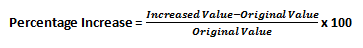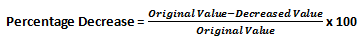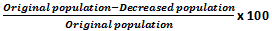# Percentage Increase Or DecreasePercentage literally means ‘per 100’, which is a number expressed as a fraction of 100. So when you say 100% of something, it means it represents the whole of it. Percentage is used to compare quantities. Let us say, you are required to find the increase or decrease in the value of a certain quantity over a period of time. Instead of quoting the numbers, this comparison can be conveniently expressed as a percentage increase or a percentage decrease in quantity. In this article, we will discuss increase or decrease as per cent.

Also Check: How to Calculate Percentage

### Percentage Increase

When comparing the increase in a quantity over a period time, we first find the difference between the original value and the increased value. We then use this difference to find the relative increase against the original value and express it in the terms of percentage. The formula for percentage increase is given by:### Percentage Decrease

When comparing the decrease in a quantity over a period of time, we first find the difference between the original value and the decreased value. We then use this difference to find the relative decrease against the original value and express it in the form of percentage. The formula for percentage decrease is given by:#### Problem Statement

1. The annual salary of Suresh increased from Rs 18,00,000 to Rs 22,00,000. Find the percentage increase.

Solution: Original salary = Rs 18,00,000

Increased salary = Rs 22,00,000

Increase in salary = Rs 22,00,000 – Rs 18,00,000 = Rs 4,00,000

Thus, percentage increase in salary = increase in salary/original salary x 100 = 4,00,000/18,00,000 x 100 = 22.22%

&;

1. The population of a small town decreased from 18,560 to 15,787 due to breakout of an epidemic. What is the percentage decrease in the population?

Solution: Original population = 18,560

Decreased population = 15,787

Decrease in population = 18,560 – 15,787 = 2773

Percentage decrease in population == 2773/18560 x 100 = 14.94%

&;